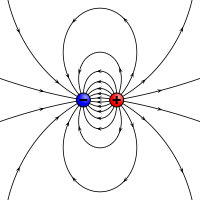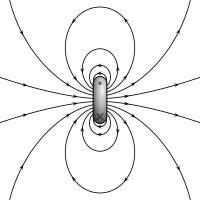# Magnetic dipole

﻿
Magnetic dipole

A magnetic dipole is the limit of either a closed loop of electric current or a pair of poles as the dimensions of the source are reduced to zero while keeping the magnetic moment constant. It is a magnetic analogue of the electric dipole, but the analogy is not complete. In particular, a magnetic monopole, the magnetic analogue of an electric charge, has never been observed. Moreover, one form of magnetic dipole moment is associated with a fundamental quantum property, the spin of elementary particles.

The magnetic field around any magnetic source looks increasingly like the field of a magnetic dipole as the distance from the source increases.

## External magnetic field produced by a magnetic dipole momentAn electrostatic analogue for a magnetic moment: two opposing charges separated by a finite distance. Each arrow represents the direction of the field vector at that point.The magnetic field of a current loop. The ring represents the current loop, which goes into the page at the x and comes out at the dot.

In classical physics, the magnetic field of a dipole is calculated as the limit of either a current loop or a pair of charges as the source shrinks to a point while keeping the magnetic moment m constant. For the current loop, this limit is most easily derived for the vector potential. Outside of the source region, this potential is (in SI units)${\mathbf{A}}({\mathbf{r}})=\frac{\mu_{0}}{4\pi}\frac{{\mathbf{m}}\times{\mathbf{r}}}{r^{3}},$

and the magnetic flux density (strength of the B-field) in teslas is$\mathbf{B}({\mathbf{r}})=\nabla\times{\mathbf{A}}=\frac{\mu_{0}}{4\pi}\left(\frac{3\mathbf{r}(\mathbf{m}\cdot\mathbf{r})}{r^{5}}-\frac{{\mathbf{m}}}{r^{3}}\right).$

Alternatively one can obtain the scalar potential first from the magnetic pole limit,$\psi({\mathbf{r}})=\frac{{\mathbf{m}}\cdot{\mathbf{r}}}{4\pi r^{3}},$

and hence the magnetic field strength (or strength of the H-field) in ampere-turns per meter is${\mathbf{H}}({\mathbf{r}})=-\nabla\psi=\frac{1}{4\pi}\left(\frac{3\mathbf{r}(\mathbf{m}\cdot\mathbf{r})}{r^{5}}-\frac{{\mathbf{m}}}{r^{3}}\right) = \mathbf{B}/\mu_0.$

The magnetic field is symmetric under rotations about the axis of the magnetic moment.

## Internal magnetic field of a dipole

The two models for a dipole (current loop and magnetic poles) give the same predictions for the magnetic field far from the source. However, inside the source region they give different predictions. The magnetic field between poles is in the opposite direction to the magnetic moment (which points from the negative charge to the positive charge), while inside a current loop it is in the same direction (see the figure to the right). Clearly, the limits of these fields must also be different as the sources shrink to zero size. This distinction only matters if the dipole limit is used to calculate fields inside a magnetic material.

If a magnetic dipole is formed by making a current loop smaller and smaller, but keeping the product of current and area constant, the limiting field is$\mathbf{B}(\mathbf{x})=\frac{\mu_0}{4\pi}\left[\frac{3\mathbf{n}(\mathbf{n}\cdot \mathbf{m})-\mathbf{m}}{|\mathbf{x}|^3} + \frac{8\pi}{3}\mathbf{m}\delta(\mathbf{x})\right].$

Unlike the expressions in the previous section, this limit is correct for the internal field of the dipole.

If a magnetic dipole is formed by taking a "north pole" and a "south pole", bringing them closer and closer together but keeping the product of magnetic pole-charge and distance constant, the limiting field is$\mathbf{H}(\mathbf{x}) =\frac{1}{4\pi}\left[\frac{3\mathbf{n}(\mathbf{n}\cdot \mathbf{m})-\mathbf{m}}{|\mathbf{x}|^3} - \frac{4\pi}{3}\mathbf{m}\delta(\mathbf{x})\right].$

These fields are related by B = μ0(H+M), where$\mathbf{M}(\mathbf{x}) = \mathbf{m}\delta(\mathbf{x})$

is the magnetization.

## Forces between two magnetic dipoles

The force F exerted by one dipole moment m1 on another m2 separated in space by a vector r can be calculated using$\mathbf{F} = -\nabla\left(\mathbf{m}_2\cdot\mathbf{B}_1\right),$

or$\mathbf{F}(\mathbf{r}, \mathbf{m}_1, \mathbf{m}_2) = \dfrac{3 \mu_0}{4 \pi r^5}\left[(\mathbf{m}_1\cdot\mathbf{r})\mathbf{m}_2 + (\mathbf{m}_2\cdot\mathbf{r})\mathbf{m}_1 + (\mathbf{m}_1\cdot\mathbf{m}_2)\mathbf{r} - \dfrac{5(\mathbf{m}_1\cdot\mathbf{r})(\mathbf{m}_2\cdot\mathbf{r})}{r^2}\mathbf{r}\right],$

where r is the distance between dipoles. The force acting on m1 is in the opposite direction.

The torque can be obtained from the formula$\boldsymbol{\tau}=\mathbf{m}_2 \times \mathbf{B}_1,$

which gives$\boldsymbol{\tau}= \frac{\mu_0}{4 \pi r^5}\left( \left(\mathbf{m}_1 \cdot \mathbf{m}_2\right) r^2 - 3(\mathbf{m}_1 \cdot \mathbf{r}) (\mathbf{m}_2 \cdot \mathbf{r}) \right).$

## Dipolar fields from finite sources

The magnetic scalar potential ψ produced by a finite source, but external to it, can be represented by a multipole expansion. Each term in the expansion is associated with a characteristic moment and a potential having a characteristic rate of decrease with distance r from the source. Monopole moments have a 1/r rate of decrease, dipole moments have a 1/r2 rate, quadrupole moments have a 1/r3 rate, and so on. The higher the order, the faster the potential drops off. Since the lowest-order term observed in magnetic sources is the dipolar term, it dominates at large distances. Therefore, at large distances any magnetic source looks like a dipole with the same magnetic moment.

Wikimedia Foundation. 2010.

### Look at other dictionaries:

• magnetic dipole — n. A dipole with opposing magnetic poles. [WordNet 1.5] …   The Collaborative International Dictionary of English

• magnetic dipole — Tiny magnet with subatomic dimensions, equivalent to the flow of electric charge around a loop. Examples include electrons circulating around atomic nuclei, rotating atomic nuclei, and single subatomic particles with spin. On a large scale, these …   Universalium

• magnetic dipole — magnetinis dipolis statusas T sritis Standartizacija ir metrologija apibrėžtis Elektros srovė, tekanti uždaru kontūru, kurio matmenys daug mažesni už atstumą tarp kontūro ir nagrinėjamojo srovės magnetinio lauko taško. atitikmenys: angl. magnetic …   Penkiakalbis aiškinamasis metrologijos terminų žodynas

• magnetic dipole — magnetinis dipolis statusas T sritis fizika atitikmenys: angl. magnetic dipole vok. magnetischer Dipol, m rus. магнитный диполь, m pranc. dipôle magnétique, m …   Fizikos terminų žodynas

• magnetic dipole — noun a dipole with opposing magnetic poles • Hypernyms: ↑dipole …   Useful english dictionary

• magnetic dipole — /mægˌnɛtɪk ˈdaɪpoʊl/ (say mag.netik duypohl) noun 1. a current loop or combination of microscopic current loops which forms a two pole magnet and tends to align itself with a magnetic field. 2. a pair of magnetic poles having equal strength and… …   Australian English dictionary

• Magnetic dipole–dipole interaction — Magnetic dipole–dipole interaction, also called dipolar coupling, refers to the direct interaction between two magnetic dipoles. The potential energy of the interaction is as follows: where ejk is a unit vector parallel to the line joining the… …   Wikipedia

• Magnetic dipole-dipole interaction — Magnetic dipole dipole interaction, also called dipolar coupling, refers to the direct interaction between two magnetic dipoles. The energy of the interaction is as follows:: old{H} = frac{ mu 0 } {4 pi r {jk}^3 } left( 3 (old{m} j cdot old{e} …   Wikipedia

• magnetic dipole moment — n. A physical quantity associated with the magnetic field of a dundamental particle; as, An orbiting electron in an atom will have a magnetic dipole moment. [WordNet 1.5] …   The Collaborative International Dictionary of English

• Magnetic dipole transition — The interaction of an electromagnetic wave with an electron bound in an atom or molecule can be described by time dependent perturbation theory. Magnetic dipole transitions describe the dominant effect of the coupling to the magnetic part of the… …   Wikipedia